• 多边形扫描转换算法。计算机图形学中一个重要的算法。该算法能够自己制定点的位置，然后进行扫描转换。
• C语言实现多边形扫描转换算法源码。 C语言实现多边形扫描转换算法，绝对原创！ C语言 多边形扫描转换 源代码 水平扫描线 扫描线
• MFC多边形扫描转换，鼠标响应生成多边形，点击进行转换 填充多边形。
• 多边形扫描转换算法（C语言实现） 原理不赘述 原理可跳转至该文章 ET边表 AET链表 实现 该算法我实在计算机图形学的书上看到了，但是遗憾的是看懂了，并没有算法实现。该算法的优势很是很明显的对于种子填充算法来...
多边形扫描转换算法（C语言实现）
原理不赘述
原理可跳转至该文章
ET边表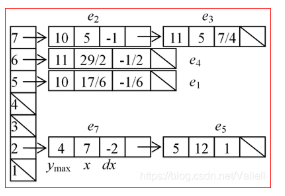AET链表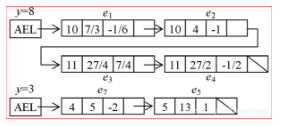实现
该算法我实在计算机图形学的书上看到了，但是遗憾的是看懂了，并没有算法实现。该算法的优势很是很明显的对于种子填充算法来说，我在电脑上用种子算法填充一个720x960的一块多边形的C语言的堆栈需要设置到32M才能够运行起来，并且填充动态肉眼可见，不用加延时就可以看到动画效果。显然时不能使用的。
这几天一直在琢磨怎么实现算法，还是纯C的那种，于是就有下面的代码。
该算法难在ET表和AET表。这里我仅仅用到了ET表，用一个简易实现串的数据结构实现的。有兴趣的朋友可以将代码复制到自己的电脑上实现一下。
使用的图形库
程序中使用到的graphics.h库大家可以访问下面的网址获取，
Easyx
使用方法请自行查阅资料和百度
#include <graphics.h>
#include <stdio.h>
/*    多边形的点数据    */
POINT points[] = { {20,10} ,{450,70}, {200,160} ,{840,500} ,{900,700} ,{640,540} ,{500,660},{400,300}, {400,510},{300,510}, {200,700} ,{100,220} };
/*    填充多边形函数 */
void draw_Polygons(POINT pointl[],int len);

int main()
{
/* 初始化图形窗口，带控制台 可以在调试中打印一些数据方便调试 */
initgraph(960, 720,EW_SHOWCONSOLE);
/* 设置坐标原点 左下角的坐标点为原点  */
setorigin(0, 719);                     //设置原点坐标
/* 设置x,y轴坐标方向 电脑是以左上角为坐标原点 至左向右为x轴正方向这里不变设置第一个参数为1，至上而下为y轴正方向，与我们所熟悉的笛卡尔坐标相反，这里设置为-1 */
setaspectratio(1, -1);                 //设置y轴正方向
/*  传入参数开始填充多边形 */
draw_Polygons(points,sizeof(points)/sizeof(POINT));
getchar();
}
/*===========================================================================
函数描述：绘制多边形
参数    ：pointl[in] 所输入的点（有序）
返回值  ：无
============================================================================*/
void draw_Polygons(POINT pointl[],int len)
{
struct toppoint             //结构体名真心不会取
{
int ymin;               //该线段最小的y值
int ymax;               //该线段最大的y值
int xmin;               //该线段最小y的对应x值
char flag;              //判断该点是否使用过
float slope;            //斜率
};
int y;
int num = 0,min,max,i,j;
//使用malloc函数可控制函数的内存大小，数组必须写死，可能会出现错误溢出等情况。
struct toppoint* ET = (struct toppoint*)malloc(len*sizeof(struct toppoint));  //申请len个边表信息;这里没有内存申请失败的处理，自觉加上
int* xstack = (int*)malloc((len + 1) * sizeof(int));  //申请xstack个点；xstack放置个数；这里没有内存申请失败的处理，自觉加上
/* 找到y的最小值 */
for (num = 0,min=pointl.y; num < len; num++)
{
if (min > pointl[num].y) min = pointl[num].y;
}
/* 找到y的最大值 */
for (num = 0, max = pointl.y; num < len; num++)
{
if (max < pointl[num].y) max = pointl[num].y;
}
//生成ET表
for (num = 0; num < len; num++)
{
ET[num].flag = 0; //没有使用过（不用数据结构去做浪费空间，要编写大量的代码删除将该标志位置一）;
ET[num].ymin = (pointl[num].y > pointl[(num + 1) % len].y ? pointl[(num + 1) % len].y : pointl[num].y);  //两者中的最小值;
ET[num].ymax = (pointl[num].y < pointl[(num + 1) % len].y ? pointl[(num + 1) % len].y : pointl[num].y);  //两者中的最大值;
ET[num].xmin = (pointl[num].y > pointl[(num + 1) % len].y ? pointl[(num + 1) % len].x : pointl[num].x);
/* 警惕除数为零的情况 */
ET[num].slope = (((float)(pointl[(num + 1) % len].y - pointl[num].y) == 0)? 0 : (float)(pointl[(num + 1) % len].x - pointl[num].x) / (float)(pointl[(num + 1) % len].y - pointl[num].y));

}
//设置填充颜色
setlinecolor(GREEN);
// 开始填充
for (y =min ; y <= max; y++)
{
// 第一个x坐标放置在 xstack的位置
i = 1;
for (num = 0; num < len; num++)   //开始推算 xstack值
{
if (ET[num].flag == 0 && y>ET[num].ymin) //没有被用过
{
if (ET[num].ymax < y)  //失效
{
ET[num].flag = 1; //已经使用过了不用再使用；
}
else
{
//利用递增的算出下一个x坐标位置
xstack[i++] = ET[num].xmin + ET[num].slope * (y - ET[num].ymin);
}
}
}
// 记录总的x坐标值个数 //均为偶数
xstack = i-1;
//开始填充 划线
// 对x坐标值进行排序
for (i = 1; i < xstack; i++)
{
for (j = 1; j < xstack + 1 -i; j++)
{
if (xstack[j] > xstack[j+1])
{
num = xstack[j];
xstack[j] = xstack[j + 1];
xstack[j + 1] = num;
}
}
}
//开始绘制该行的线段
for (num = 1; num <= xstack; num += 2)
{
line(xstack[num], y, xstack[num + 1], y);
}
}
//设置边的颜色值
setlinecolor(RED);
for (num = 0; num <= len; num++)
{
// 画线
line(pointl[num % len].x, pointl[num % len].y, pointl[(num + 1) % len].x, pointl[(num + 1) % len].y);
}
}

效果展示：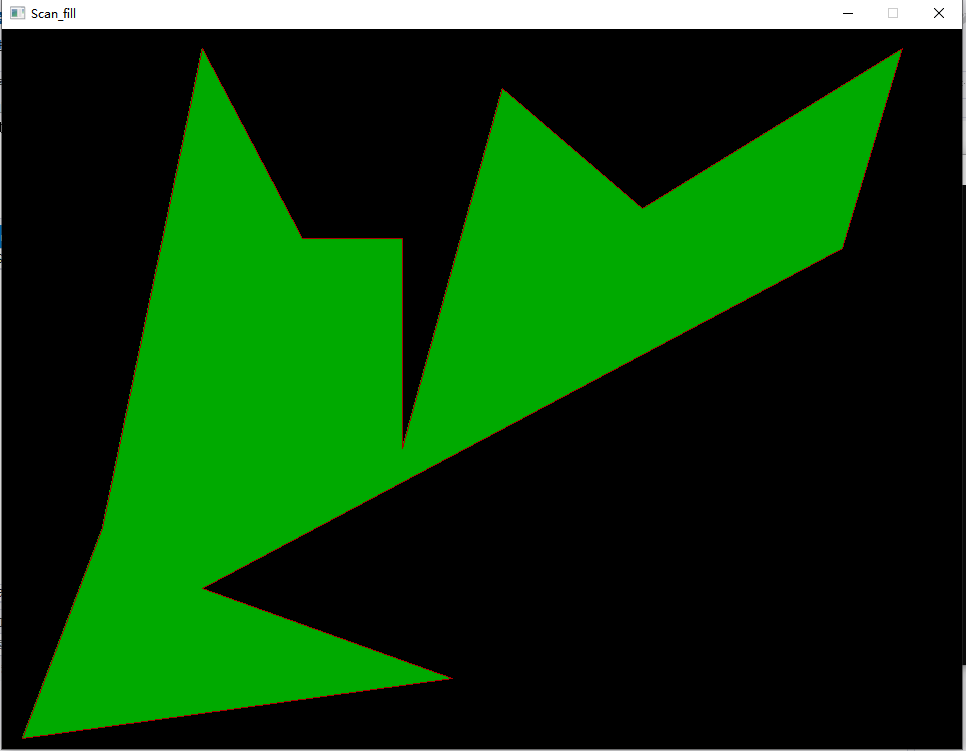展开全文• 实现任意一个非自交五边形的扫描转换（要求采用扫描线算法
• 多边形扫描转换算法效果代码 效果 首先点击几个点作为多边形顶点，按下“e”画出多边形轮廓。接下来按下“r”、“g”、“b”三个按键中的任一个选择对应颜色填充多边形。它会慢慢自上往下填充。 这里说明一下，...
多边形扫描转换算法效果代码
效果
首先点击几个点作为多边形顶点，按下“e”画出多边形轮廓。接下来按下“r”、“g”、“b”三个按键中的任一个选择对应颜色填充多边形。它会慢慢自上往下填充。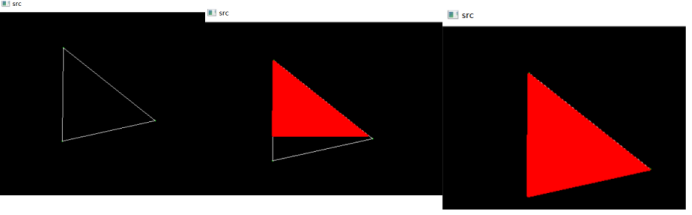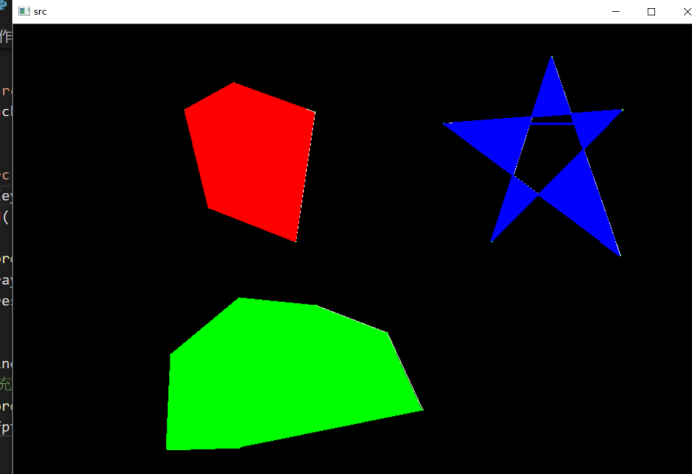这里说明一下，代码可能还有点小问题，边数多了会填充出错
代码
import cv2
import numpy as np
import time

img  =  np.zeros((700, 900, 3), np.uint8)#背景
pts=[]

def draw_func(event, x, y, flags, param):
global pts
if event == cv2.EVENT_LBUTTONDOWN:
print('(x:',x,',y:',y,')')
str1 = '(x:'+ str(x) + ',y:'+ str(y) + ')'
#cv2.putText(img,str1 , (x, y), cv2.FONT_HERSHEY_PLAIN,1.0, (0, 0, 255), thickness=1)
cv2.circle(img,(x,y),1,(0,255,0),thickness=1)
pts.append([x,y])

#活性边表与新边表中的节点
class Node:
def __init__(self,xmin,ymax,k1,next=None):
self.x=xmin#也就是x的初始值
self.ymax=ymax
self.k1=k1#也就是δx
self.next=next
def print(self):
t=self
while t!=None:
print(t.x,t.ymax,t.k1,end="-->")
t=t.next
print()

def FillPoly(fpts,color):
#cv2.fillPoly(img,fpts,color)
Ymin,Ymax=1000000,0
for pt in fpts:
if Ymin>pt:
Ymin=pt
if Ymax<pt:
Ymax=pt

#构造新边表
NET=[x for x in range(Ymax+1)]
for i in range(len(fpts)):
#后面一个点的序号
j=i+1 if i<len(fpts)-1 else 0
if fpts[i]>fpts[j]:
ymax,ymin=fpts[i],fpts[j]
xmin=fpts[j]
else:
ymin,ymax=fpts[i],fpts[j]
xmin=fpts[i]
#与x轴平行,跳过该边
if fpts[i]==fpts[j]:
continue

if fpts[i]==fpts[j]:
k1=0
else:
k1=(fpts[i]-fpts[j])/(fpts[i]-fpts[j])

if type(NET[ymin])!=type(66):
t=NET[ymin]
while t.next!=None:
t=t.next
t.next=Node(xmin,ymax,k1)
else:
NET[ymin]=Node(xmin,ymax,k1)
for i,x in enumerate(NET):
if type(x)!=type(66):
print(i)
x.print()

AET=Node(0,0,0)
print("YY")
for y in range(Ymin,Ymax+1):
print(y)
#慢慢画
cv2.waitKey(3)
cv2.imshow('src',img)
#加入新边
if type(NET[y])!=type(66):
t=AET
while t.next:
t=t.next
t.next=NET[y]
#填充颜色
#按x排序
xs=[]
t=AET
while t.next:
t=t.next
xs.append(t.x)
xs=sorted(xs)
for i in range(1,len(xs),2):
for x in range(int(xs[i-1]),int(xs[i])):
cv2.circle(img,(x,y),1,color,thickness=1)
#删除并更新边
t=AET
while t.next:
ct=t.next
ct.x+=ct.k1
if ct.ymax==y:
t.next=ct.next
t=t.next
if t==None:
break

cv2.namedWindow('src')
cv2.setMouseCallback('src',draw_func)

while(1):
cv2.imshow('src',img)
code=cv2.waitKey(100)
if code == ord('q'):#按下q退出
break
elif code == ord('e'):#按下该键表示画完一个多边形
tmp=np.array(pts,np.int32)
tmp=[tmp.reshape((-1,1,2))]
fpts=pts
pts=[]
cv2.polylines(img,tmp,True,(255,255,255))
#按下 r,g,b 填充多边形为相应的颜色
elif code == ord('r'):
FillPoly(fpts,(0,0,255))
elif code == ord('g'):
FillPoly(fpts,(0,255,0))
elif code == ord('b'):
FillPoly(fpts,(255,0,0))
cv2.destroyAllWindows()




展开全文python
• 一、多边形扫描转换算法——X扫描线算法 1. 背景 1° 多边形的两种重要表示方法：顶点表示和点阵表示； 2° 光栅图形的一个基本问题就是把多边形的顶点表示转换成点阵表示。称为多边形的扫描转换； 2. 原理 X...
一、多边形扫描转换算法——X扫描线算法
1. 背景
1°  多边形的两种重要表示方法：顶点表示和点阵表示；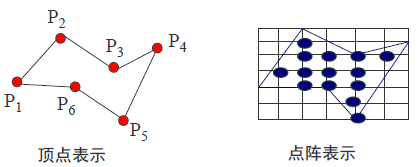2° 光栅图形的一个基本问题就是把多边形的顶点表示转换成点阵表示。称为多边形的扫描转换；
2. 原理
X-扫描线算法填充多边形的基本思想是按扫描线顺序，计算扫描线与多边形的相交区间，再用要求的颜色显示这些区间的像素，即完成填充工作；区间的端点可以通过计算扫描线与多边形边界线的交点获得。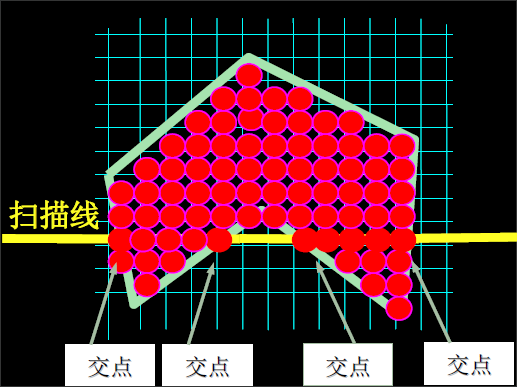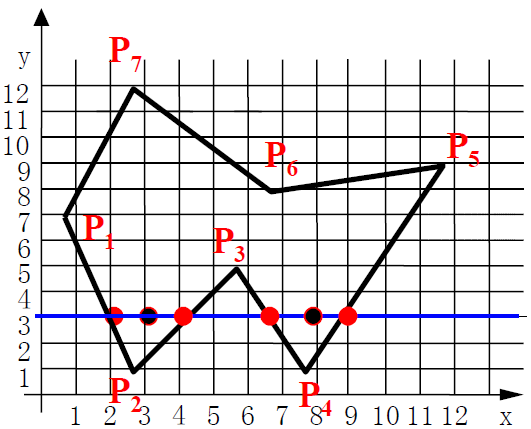如扫描线y=3与多边形的便捷相交于4点：（2,3）、（4,3）、（7,3）、（9,3）；
这四点定义了扫描线上的区间（2,4）、（7,9），区间内像素应取填充色；
算法核心：按X递增顺序排列交点的X坐标序列。
3. 算法步骤
1° 确定多边形所占有的最大扫描线书，得到多边形顶点的最小和最大Y值（ymin、ymax）;
2° 从y = ymin到y = ymax，每次用一条扫描线进行填充；
3° 对一条扫描线填充的过程可分为四个步骤：
a. 求交：计算扫描线与多边形各边的交点；
b. 排序：把所有交点按递增顺序进行排序；
c. 交点配对：第一个与第二个，第三个与第四个；
d. 区间填色：把这些相交区间的像素置成不同于背景色的填充色；
4. 交点取舍问题  ---->  交点的个数应保证为偶数个
a. 若共享顶点的两条边分别落在扫描线的两边，交点只算一个；
b. 若共享顶点的两条边在扫描线的同一边，这时交点作为零个或两个；
【注意】检查共享顶点的两条边的另外两个两个端点的y值，按这个y值中大于交点y值的个数来决定交点数；（大于-->2、小于--->0）
5. 求交
为了计算每条扫描线与多边形的交点，最简单的方法是把多边形的所有边放在一个表中。在处理每条扫描线是，按顺序从表中取出所有的边，分别与扫描线求交；计算量非常大！！！
二、多边形扫描转换算法——改进的X扫描线算法
1. 引入特殊的数据结构
1）活性边表（AET）：把当前扫描线相交的边成为活性边，并把它们按与扫描线交点x坐标递增的顺序存放在一个链表中；
2）结点内容（一个结点在数据结构里可用结构来表示）
x：当前扫描线与边的交点坐标
∆x：从当前扫描线到下一条扫描线间x的增量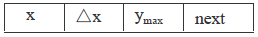ymax：该边所交的最高扫描线的坐标值ymax
3）新边表（NET）：为了方便活性边表的建立与更新，用来存放多边形的边的信息
a）首先构造一个纵向链表，链表的长度为多边形所占有的最大扫描线数，链表的每个结点，成为一个吊桶，对应多边形覆盖的每一条扫描线；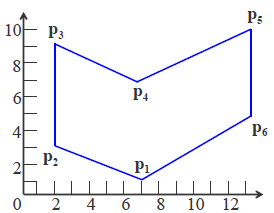====》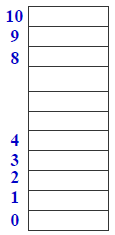b）NET挂在与该边低端y值相同的线桶中。也就是说，存放在该扫描线第一次出现的边；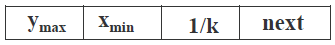数据结构内容：该边的ymax、该边较低点的x坐标值xmin，该边的斜率1/k、指向下一条具有相同较低端y坐标的边的指针；
示例：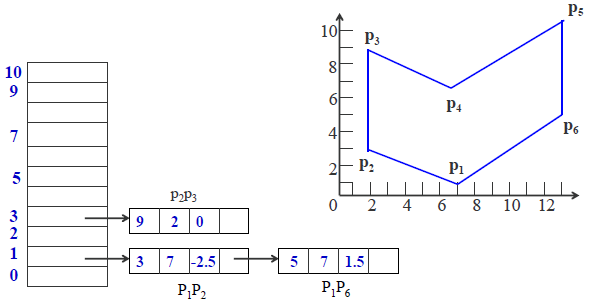2. 算法原理
1° 随着扫描线的移动，扫描线与多边形的交点和上一次交点相关：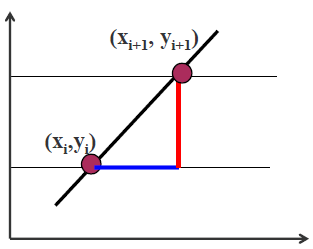设边的直线斜率为k：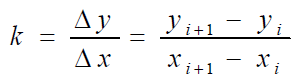推导得：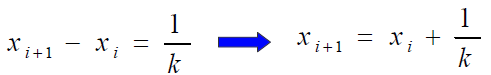，即∆x = 1 / k；
2° 需要知道一条边何时不再与下一条扫描线相交，以便及时把它从有效边表中删除出去，避免下一步进行无谓的计算；ymax是边所在的最大扫描线值，通过它可以知道何时才能“抛弃”该边。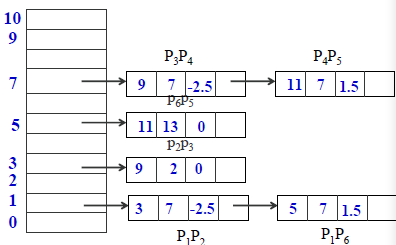从上边这个NET表里就知道多边形是从哪里开始的：在这个表里只有1、3、5、7处有边，从y=1开始做，而在1这条线上有两条边进来了，然后就把这两条边放进活性边表来处理。
3° 每一次更新，需要对已有的边进行三个处理：
1）是否被去除掉；
2）如果不被去除，第二就要对它的数据进行更新。所谓更新数据就是要更新它的x值，即x+1/k;
3）看有没有新的边进来，新的边在NET里，可以插入排序插进来；
转载于:https://www.cnblogs.com/mzyan/p/9704008.html
展开全文• X-扫描转换算法效率低下，主要原因时求交。为避免求交运算，需引进特殊得数据结构。 数据结构： 1、活性边表（AET）：把与当前扫描线相交得边称为活性边，并把它们按与扫描线交点x坐标递增得顺序存放在一个链表中。 ...
X-扫描转换算法效率低下，主要原因时求交。为避免求交运算，需引进特殊得数据结构。
可以从三个方面考虑加以改进：
1、在处理一条扫描线时，仅对与它相交的多边形的边（有效边）进行求交运算
2、考虑扫描线的连贯性：即当前扫描线与各边的交点顺序与下一条扫描线与各边的交点顺序很可能相同或非常相似
3、多边形的连贯性：即当某条边与扫描线相交时，它很可能也与下一条扫描线相交

数据结构：
1、活性边表（AET）：把与当前扫描线相交得边称为活性边，并把它们按与扫描线交点x坐标递增得顺序存放在一个链表中。
2、结点内容（一个结点在数据结构里可用结构来表示）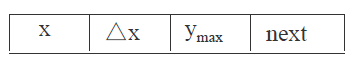x：当前扫描线与边得交点坐标
Δx：从当前扫描线到下一条扫描线间x得增量
ymax：该边所交的最高扫描线的坐标值ymax
next：指向下一个结点

随着扫描线的移动，扫描线与多边形的交点和上一次交点相关：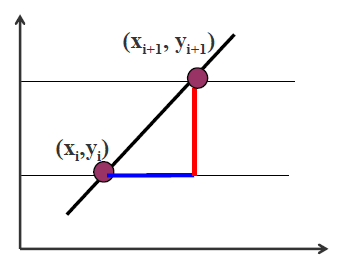设边的直线斜率为k：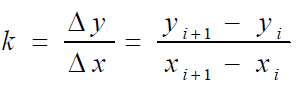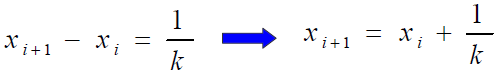即Δx=1/k
另外，需要知道一条边何时不再与下一条扫描线相交，以便及时把它从有效边表中删除出去，避免下一步进行无谓的计算
例：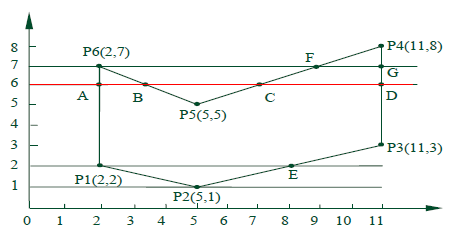此时活性链表为：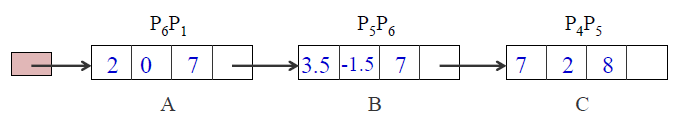为了方便活性边表的建立与更新，需构造一个新边表（NET），用来存放多边形的边的信息：
1、首先构造一个纵向链表，链表的长度为多边形所占有的最大扫描线数，链表的每个结点称为一个吊桶，对应多边形覆盖的每一条扫描线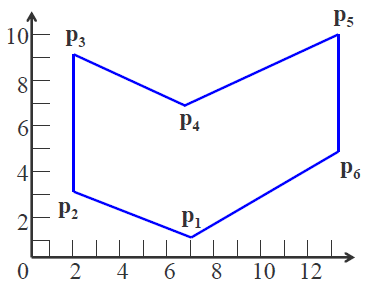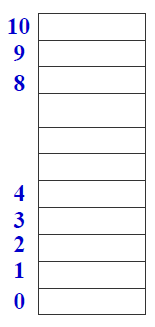2、NET挂在与该边最低端y值相同的扫描线桶中。即存放在该扫描线第一次出现的边ymax：该边最大的y值
xmin：该边较低点的x坐标值
1/k：该边的斜率
next：指向下一条具有相同较低端y坐标的边的指针
上图的NET为：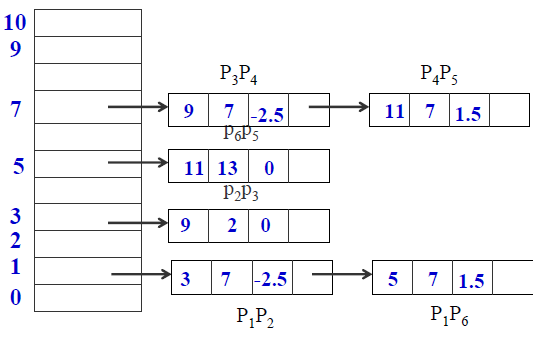从这个NET表里可知多边形是从哪里开始的，在这个表中只有1，3，5，7处有边，从y=1开始做，而在1这条线上有两条边进来了，然后就把这两条边放进活性边表来处理
每做一次新的扫描线时，要对已有的边进行三个处理：
1、是否被去除掉
2、如果不被去除，第二就要对它的数据进行更新。所谓更新数据就是更新x值，即：x+1 / k
3、看是否有新的边进来，新的边在NET里，可插入排序插进来void polyfill (polygon, color)
int color; 多边形polygon;
{ for (各条扫描线i )
{ 初始化新边表头指针NET[i]；
把ymin = i 的边放进边表NET[i];
}
y = 最低扫描线号；
初始化活性边表AET为空；
for (各条扫描线i )
{
把新边表NET[i] 中的边结点用插入排序法插入AET表，使之按x坐标递增顺序排列；
遍历AET表，把配对交点区间(左闭右开)上的象素(x,y)，用putpixel(x,y,color) 改写象素颜色值；
遍历AET表，把ymax= i 的结点从AET表中删除，并把ymax>i
结点的x值递增x；
若允许多边形的边自相交，则用冒泡排序法对AET表重新排序；
}
} /* polyfill */多边形扫描转换算法的缺点时无法实现对未知边界的区域填充

展开全文• 这个算法其实很复杂的，实现起来需要有耐心，一步一步按照算法思路来写代码。 在算法中，我使用的是静态链表（c#没有指针-_-||） 下面的AET为活性边表，NET存储新边表 1.定义数组，变量 class AET//定义AET表，不论...计算机图形学
• 2.扫描算法画直线的时候，可能同一行有多个点相邻的情况，如果遇到这样的点就变号结果会出现错误。 3.两条相邻边的路径可能经过同一个点，尤其是dy&amp;amp;gt;&amp;amp;gt;dx的时候，不加以判断还是会出错...计算机图形学
• class ChainNode {//node class Object node; ChainNode next=null; ChainNode(){} ChainNode(Object element) { this.node=element; ... ChainNode(Object element,ChainNode next) ...
• 我们在初始化时建立一个全局的边表(ET),它包含多个，它包含多边形的所有边，并且这些边按照它们各自y坐标较小值排序。 一般地，ET是基于桶排序的方式建立的，有多少扫描线就有多少个桶。在每个桶中，根据边的较低的...
• 活性边表(Active Edge Table, AET) 在上一节中，我们讲解了如何生成根据多边形生成ET 这一节我们讲解活性边和活性边表。 首先，什么是活性边？...它记录了多边形边沿扫描线的交点序列。 一旦生成了ET, 扫...
• 用VC++完成的多边形扫描转换算法，对多边形内部节点进行扫描
• 1. 目的：输入多边形顶点，对多边形进行填充，使用多边形扫描转换算法； 2. 实现： const int POINTNUM=6; //多边形点数. /******定义结构体用于活性边表AET和新边表NET***********************************/ ...
• 2．通过C++语言编程实现多边形扫描转换的扫描线填充算法 多边形分类：可分为凸多边形、凹多边形、含内环多边形。 （1）凸多边形：任意两顶点间的连线均在多边形内。 （2）凹多边形：任意两顶点间的连线有不在多边形...
• PAGE / NUMPAGES 贵州大学计算机图形学实验报告 学院计算机科学与信息学院 专业软件工程 班级 姓名 学号 实验组 实验时间 指导教师 成绩 实验项目名称 实验三 多边形扫描转换算法区域填充算法 实验目的 通过本实验...
• 采用VC++语言编程实现多边形扫描转换的扫描线填充算法，可以画任意多边形。 多边形为任意多边形，如：凸多边形、凹多边形、含内环多边形。 上传的资源中没有采用通过鼠标画来实现多边形顶点的输入，而是在代码中人...
• OpenGL实现多边形扫描转换的扫描线算法，带动画效果 实验作业，LAB3. 绘制的是五边形。
• 扫描线算法扫描转换多边形的常用算法，它充分利用了相邻像素之间的连贯性，避免了逐点判断和反复求交计算，达到了减少计算量和提高算法效率的目的。 处理对象：非自交多边形 （边与边之间除了顶点外无其它交点）...
• 多边形扫描转换功能，可以用于3D背景剔除，CPU扫描转换ZBUFFER算法...